Home  |  Contact
 DESENMASCARANDO LAS FALSAS DOCTRINAS

 What’s NewMessage Board
Image Gallery
Files and Documents
Polls and Test
Member List
YHWH (DIOS PADRE) EL UNICO DIOS
JESUCRISTO NUESTRO MESIAS JUDIO
LOS DIEZ MANDAMIENTOS DE LA BIBLIA
MEJORE SU CARACTER Y SU VIDA
BIBLIAS/CONCORDANCIA/LIBROS
MAYOR ENEMIGO DEL HOMBRE ES UNO MISMO
¿LA TORA ES MACHISTA? -MENSAJE ESOTERICO Y EXOTERICO
¿ES INMORTAL EL ALMA?- FALACIA DE LA ENCARNACION Y REENCARNACION
EL ISLAM TIENE ORIGEN UNITARIO ADOPCIONISTA
ANTIGUO TESTAMENTO-ESTUDIO POR VERSICULOS
NUEVO TESTAMENTO-ESTUDIOS POR VERSICULOS
NUEVO TESTAMENTO II-ESTUDIOS POR VERSICULOS
NUEVO TESTAMENTO III-ESTUDIOS POR VERSICULOS
CRISTO NO TUVO PREEXISTENCIA
¿QUE ES EL ESPIRITU SANTO?

Tools

General: Sacred Geometry and Vedic Mathematics
Choose another message boardPrevious subject  Next subjectReply Message 1 of 102 on the subject
 From: BARILOCHENSE6999  (Original message) Sent: 03/11/2020 13:37
 https://www.jain108.com/FirstPrevious  88 to 102 of 102  NextLastReply Message 88 of 102 on the subject
 From: BARILOCHENSE6999 Sent: 25/07/2021 22:53Reply Message 89 of 102 on the subject
 From: BARILOCHENSE6999 Sent: 25/07/2021 23:11
 “e” = 2.718281828459045… JAIN 108 NEW FORMULA for the EXPONENTIAL FUNCTION Jain 108 discovered a better way for defining the formula for “e” = 2.718281828459045 etc.This is the Exponential Number for nature’s growth and decay. “e” is the mathematical constant that measures the growth of human populations or viral colonies. It also is used in Compound Interest formulae, in Physics, it is used to establish the half-life of an element by studying its radioactive decay, and is used by coroners to predict the time of someone’s death for murder investigations. It appears to act like the Phi Ratio, connected to biological systems, but is another separate entity, and is as important.I first Published this Discovery briefly in “In The Next Dimension” aka The Book of Phi, Volume 2, by Jain in 2003 but was received in 2000.In other Discoveries:Jain independently revealed the Infinitely Repeating 24 Pattern in the Fibonacci Sequence, by continued subtraction of 9 aka Digital Roots or Digital Compression. He also discovered 2 important codes in the Wheel of 60 revealed when we continually subtract 10 from the Fibonacci Sequence, which is really observing the final or end digits, to show that a spiral pattern of these 60 infinitely repeating digits actually reEnters itSelf precisely on the 60th digit, and also that there is a hidden Harmonic 1111 and 6666 code embedded in the Wheel of 60… see the articles on this on this site. There is great practical applicability to this Fibonacci 60 Code because Tesla knew that the 60 hertz or cycles per second in our electrical world is the most conducive for the human condition.The Pervasiveness of Phi (1.618033988…). Jain can mathematical show that Phi is in all important systems, in biology, in crystals, in space, in atoms, and mathematically it is seen everywhere in the Doubling Binary Sequence (1-2-4-8-16-32-64), in the Pythagorean 3-4-5 Triangle, surprisingly in the Equilateral Triangle, in Magic Squares, in Prime Number Sequences etc.C=2.718281728…UPDATE 1 on “e: Jain’s Discovery” Validated by Ewan Donovan“e’ has now been VALIDATED ! In my Instagram movie (igtv) I made a call to get assistance by computer-savvy technicians to improve the number of decimal places in e using my new formula.“e’ has now been confirmed for n =3,000,000 or 3 millionThanks to Ewan Donovan of the UK who sent me the latest update: e = 2.7182818284590…correct to  13 dp. (26th July, 2019)Previously, I had recorded n= 2,800 to get e precise to 7 decimal places: e= 2.7182818…to 7 decimal places. Then Zevan Rosser‘s responded on my Facebook Business Page Jain 108 Mathemagics, improving “e” to 2 more decimal places, by plugging in n=20,000 giving the correct value of 2.718281828…which is 9 dp (decimal places).Then  Anthony Canosa, plugs in n=100,000 into my new formula and advances the 9 dp to 10 dp with the value of e=2.7182818284…Observe, that it only advanced 1 dp, from going from n=20,000 to n=100,000.It appears, that we have to plug in n=1 billion and 1 trillion.e = 2.718281828459045235…  this is e correct to 18 dp as found in mathematical textbooks.Jain 108   (July 2019)https://jain108academy.com/e-2-718281828459045-jain-108-new-formula-for-the-exponential-function/

 Reply Message 90 of 102 on the subject
 From: BARILOCHENSE6999 Sent: 25/07/2021 23:25Testimonial by Kylie Davidson who predicts that this new e Formula is somehow connected to Gravity and Earth Grid Correctional Codes.There is a special relationship between the  3 top mathematical constants known as Phi, Pi and the Exponential Function. They are shown here to be involved with the Pythagorean 3-4-5 Triangle, but the mathematics to prove this is only 99.9% correct.Thanks to Scott Onstott who offered this brilliant connection to e regarding the ratio volume of a sphere and a cube! If you would like to have this 16 page article as a PDF, and contribute some feedback regarding this New Formula, please contact me via email on jain@jain108.com.Thanks for your time reading this. PDF OF JAIN NEW E FORMULA 16 PAGES Here is the 15 minute video of this e article, that was originally first shown on our Instagram page called @108academy. C=2.718281728…UPDATE 1 on “e: Jain’s Discovery” Validated by Ewan Donovan “e’ has now been VALIDATED !In my Instagram movie (igtv) I made a call to get assistance by computer-savvy technicians to improve the number of decimal places in e using my new formula.“e’ has now been confirmed for n =3,000,000 or 3 millionThanks to Ewan Donovan of the UK who sent me the latest update: e = 2.7182818284590…correct to  13 dp. (26th July, 2019)Previously, I had recorded n= 2,800 to get e precise to 7 decimal places:  e= 2.7182818…to 7 decimal places  .Then Zevan Rosser‘s responded on my Facebook Business Page Jain 108 Mathemagics, improving “e” to 2 more decimal places, by plugging in n=20,000 giving the correct value of 2.718281828…which is 9 dp (decimal places).Then Anthony Canosa, plugs in n=100,000 into my new formula and advances the 9 dp to 10 dp with the value of e=2.7182818284…Observe, that it only advanced 1 dp, from going from n=20,000 to n=100,000.It appears, that we have to plug in n=1 billion and 1 trillion.e = 2.718281828459045235…  this is e correct to 18 dp as found in mathematical textbooks.Jain 108      (July 2019)UPDATE 2 on “e: Jain’s Discovery” with Acknowledgement to H.J. Brothers and J.A. Knox I would like to express that one reader claimed that he scoured the internet and on Wikipedia he found the so called Jain “e” formula (published by Jain in 2002 but realized around 2000), in another 1998 journal called “The Mathematical Intelligencer, Vol 20, No. 4, (1998), pp. 25-29”. It had a name called:“e the Symmetric Limit”.Citation  H.J. Brothers and J.A. Knox, New closed-form approximations to the Logarithmic Constant e. In this regard, I humbly acknowledge that indeed the 2 mathematicians Brothers and Knox did publish the same formula that I had independently realized, so credit must go to them, but I claim that I had no knowledge of this journal, that also published 7 other different ways of achieving “e” to high decimal accuracy, that I had not seen this in print anywhere, thinking that there was only one traditional way of deriving “e’ via Euler’s fractional factorials. In the article I read, it said that the first evidence of “e” was by Joost Burgi in 1620 correct to 3 decimal places, before Newton had published his version in 1669.The reader from Instagram had a code name of: Arrebarritra, this is what he kindly sent:The next 2 images below are taken from Wikipedia:(refer to Note no.7 above) Just for the reader’s reference, I am including the first 2 pages of the “7 New Ways of Deriving e” as published in the Mathematical Intelligencer in 1928, to show that great minds are at work here, and it is a pleasure to acknowledge them, and consider myself as one of these researchers. The second page shown here does not show the one that I discovered, there are meant to be a few more pages but they were not released on the internet unless you became a subscriber to the magazine; it is included just to show the mathematical depth of the article.I would like to conclude that the lesson I have learnt here is not to assume that you have discovered anything, since the age of the Internet, there exist many like Minds, all who believe they have independently Cracked A Code, not realizing that this is Universal Knowledge, and that all we are really doing is ReMembering this lost Knowledge. If a mathematician needs to have their name to a discovery, it is safe to call it a “Re-Discovery” and without being criticized as having a great Ego, it is understood that such self-proclaimed mathematicians are genuine questors for this mysterious symmetry, and by having their name attached to an assumed Discovery is merely an acknowledgement that they have devoted their time and lifeforce in the pursuit of the evasive Laws of Numbers. (When I studied Professional Writing at university in my early years, my teacher insisted that we always acknowledge the source of any sentences or paragraphs or quotes designed or penned by others, expressing that in the event that the quoted material was in error, then this act of quoting their name to the source actually protects the writer. Thus it is our duty to always quote the source of our material and not just paraphrase it with the intent of claiming someone else’s original work as our own. In this light, I acknowledge the “e” discovery that I thought I had discovered as the intellectual realization of H.J. Brothers and J.A. Knox.Regards, Jain23-8-2019. Mullumbimby Creek. https://www.jain108.com/e-2-718281828454590/

 Reply Message 91 of 102 on the subject
 From: BARILOCHENSE6999 Sent: 21/08/2021 01:40

 Reply Message 92 of 102 on the subject
 From: BARILOCHENSE6999 Sent: 22/08/2021 02:14Reply Message 93 of 102 on the subject
 From: BARILOCHENSE6999 Sent: 29/08/2021 00:56
 http://www.circleissquared.com/

 Reply Message 94 of 102 on the subject
 From: BARILOCHENSE6999 Sent: 30/08/2021 23:37
 (1) I begin the demonstration with a typical set of nested squares & circles. This diagram is very easy to construct with unmarked compass & straightedge, so I won’t bother with extraneous details. Although measurement is not used to construct these figures, it is obviously required for the proof. Therefore, I will treat the innermost Green Circle as a Unit Circle; (Radius = 1, Area = Pi). The outer Blue Circle is twice the size of the Green Circle; (Radius = √2, Area = 2Pi). Also, you will notice that I’ve labeled key intersecting points with the letters A-E, and made two line extensions; one in the center & one at the bottom. These will come into play in succeeding diagrams. http://www.circleissquared.com/

 Reply Message 95 of 102 on the subject
 From: BARILOCHENSE6999 Sent: 09/09/2021 00:27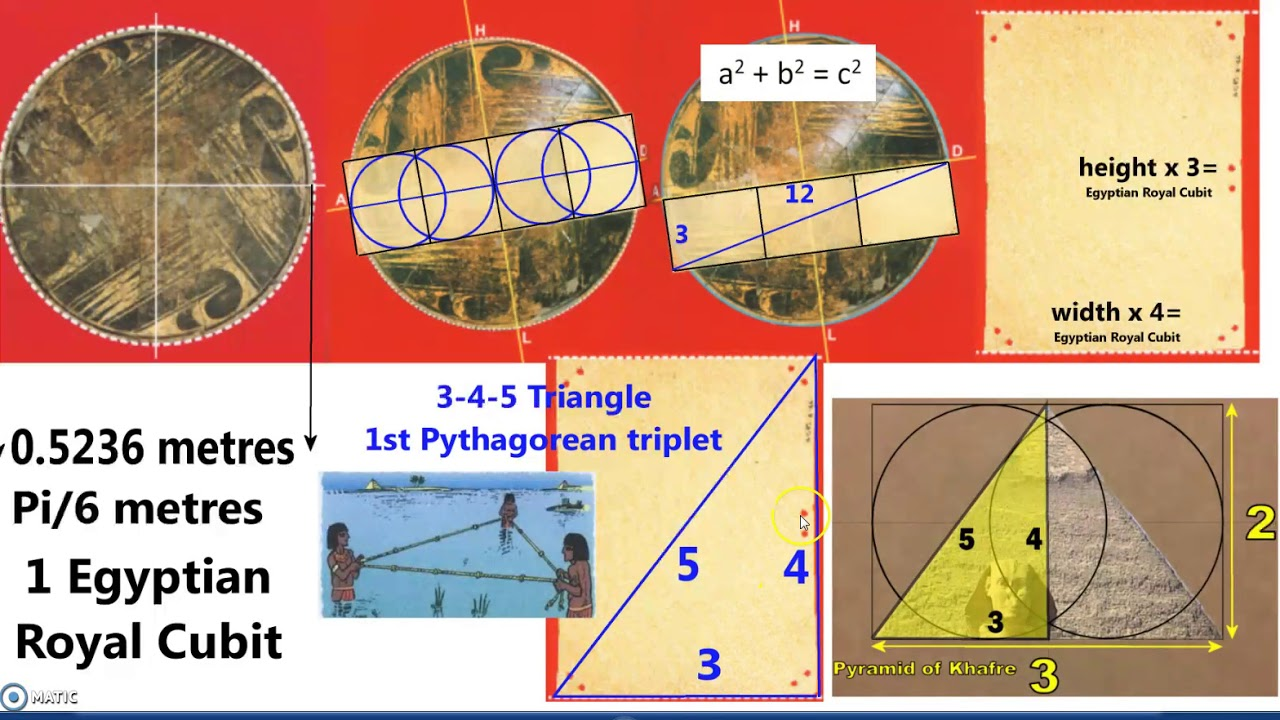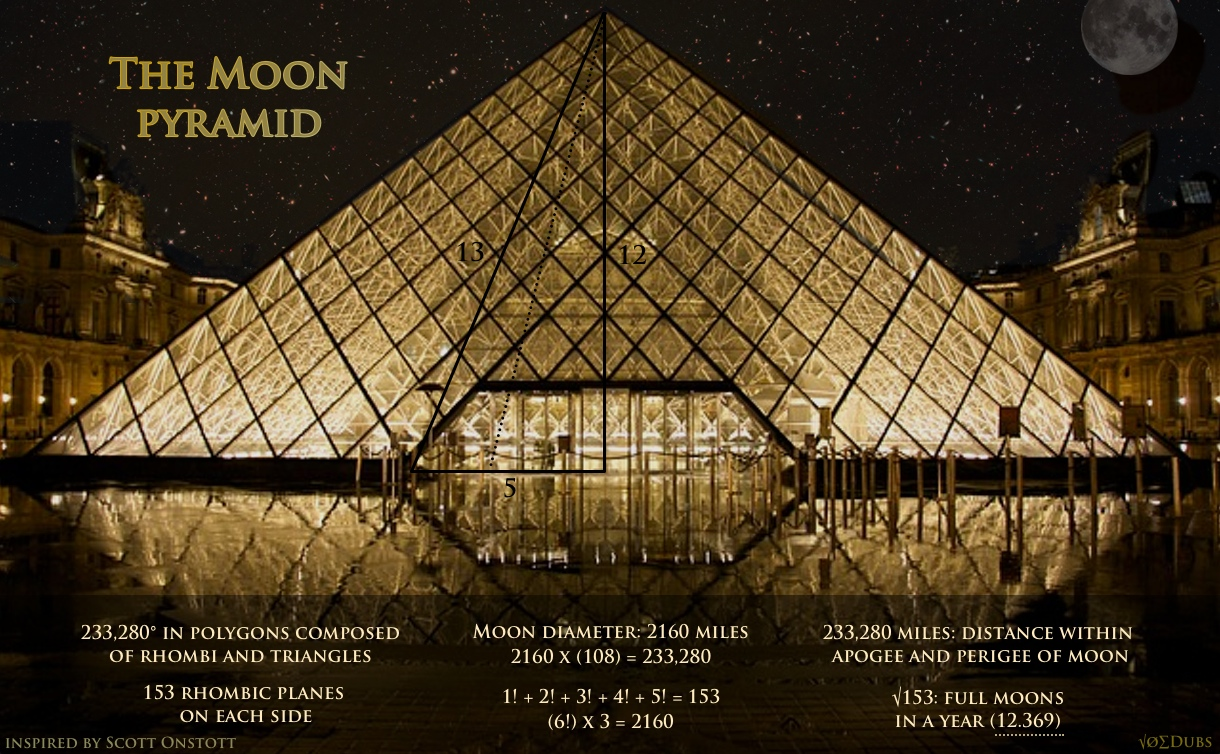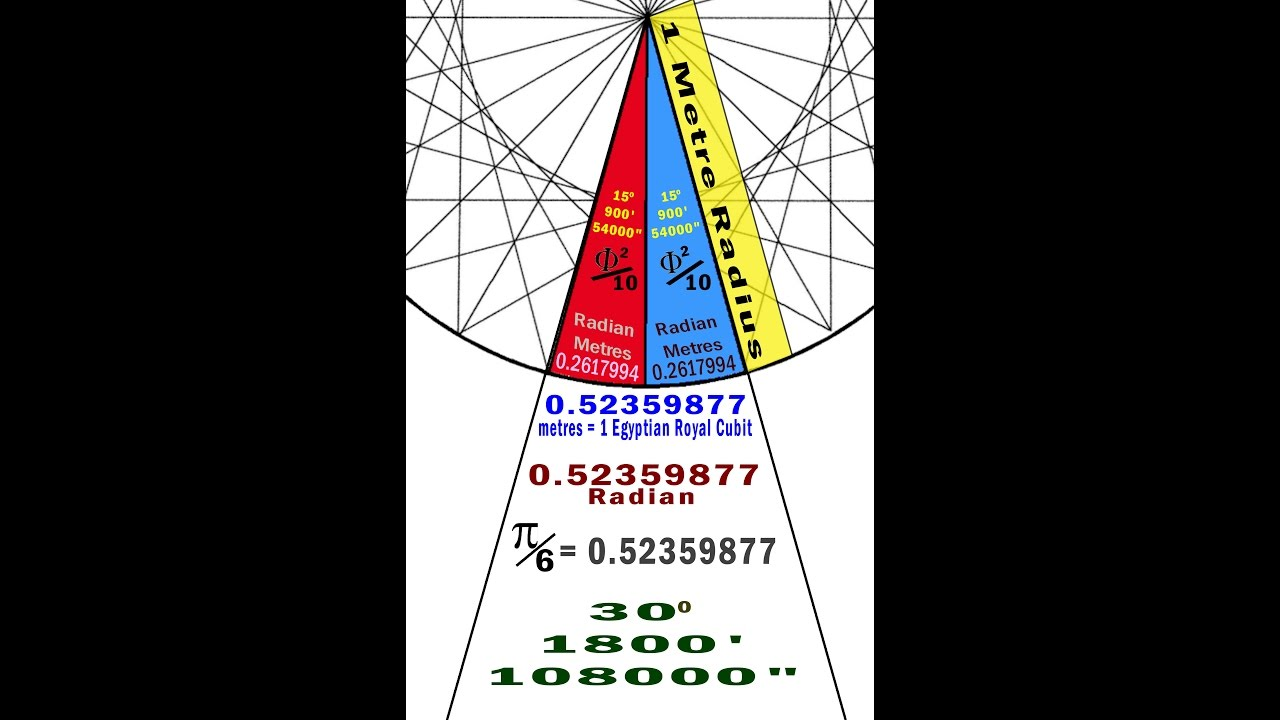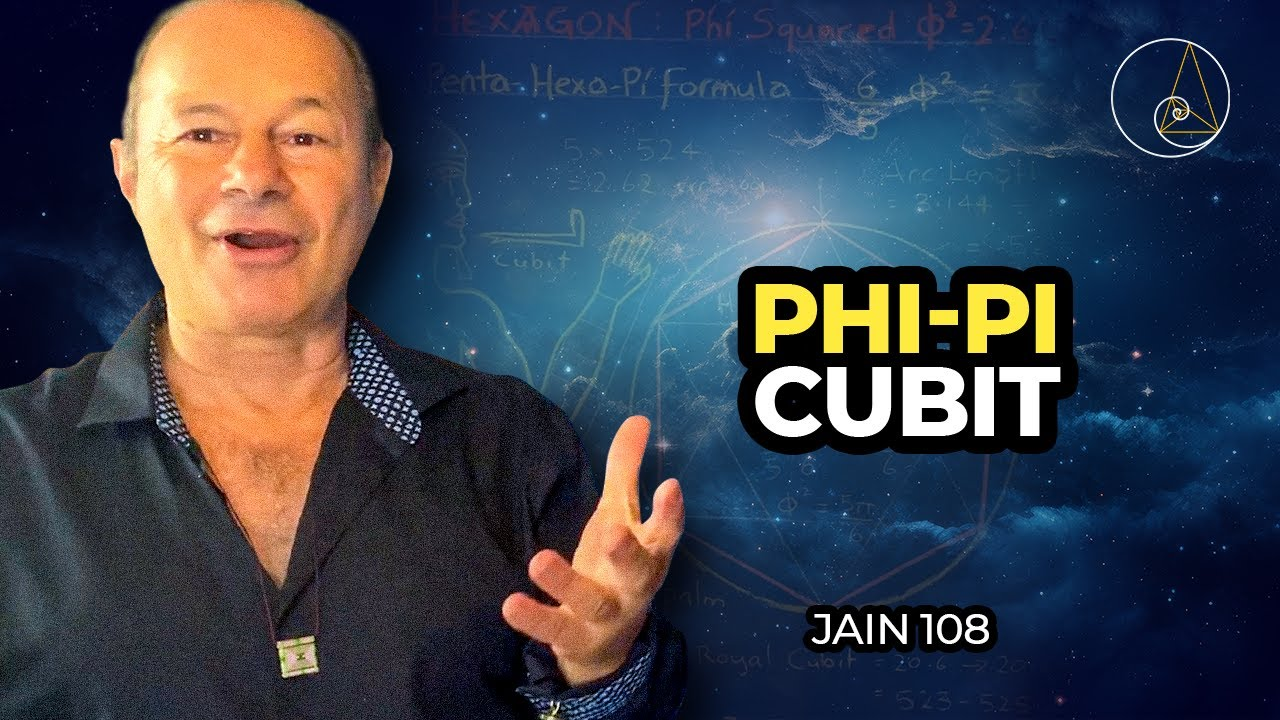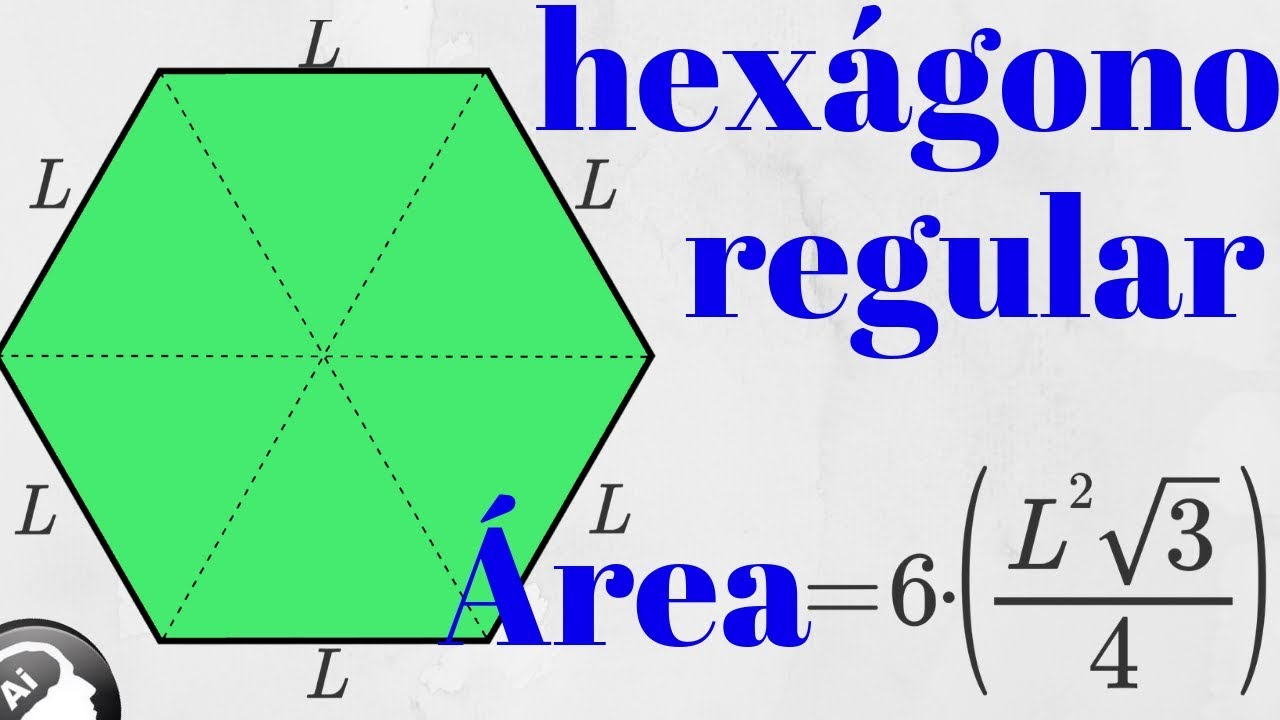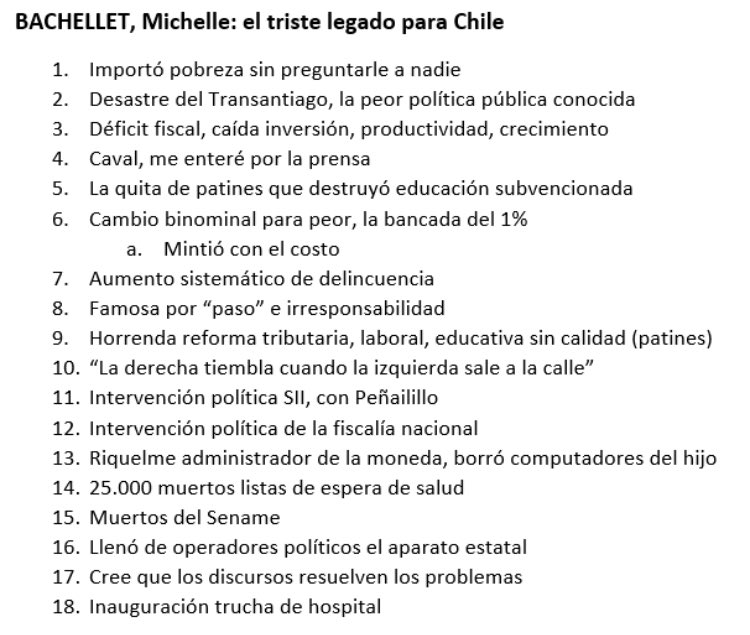Reply Message 96 of 102 on the subject
 From: BARILOCHENSE6999 Sent: 09/09/2021 00:47Reply Message 97 of 102 on the subject
 From: BARILOCHENSE6999 Sent: 09/09/2021 00:50Reply Message 98 of 102 on the subject
 From: BARILOCHENSE6999 Sent: 11/09/2021 19:42

 Reply Message 99 of 102 on the subject
 From: BARILOCHENSE6999 Sent: 16/09/2021 02:14

 Reply Message 100 of 102 on the subject
 From: BARILOCHENSE6999 Sent: 16/09/2021 23:05
 The amount of days in a solar year plus the proportions for the Equatorial circumference of the Earth and the proportions of the Great Pyramid of Giza according to Golden Pi = 4/√φ = 3.144605511029693144: The Great Pyramid of Giza is a geodetic model of Planet Earth. The measurements mentioned below are the ideal measurements. A meter is equal to 100 centimeters 1 Solon cubit = 40 times √φ = 50.88078598056276 centimeters. If 1 Solon cubit is divided into 20 equal units of measure then 20 inches can be derived because 1 Solon cubit is equal to 20 inches. 1 Saylen cubit = 50 times √φ = 63.60098247570345 centimeters. If 1 Saylen cubit is divided into 25 equal units of measure then 25 inches can be derived because 1 Saylen cubit is equal to 25 inches. 1 inch = 2 times √φ = 2.544039299028138 centimeters. 1 foot = 12 inches. 1 foot = 24 times √φ = 30.528471588337656 centimeters. If the shortest edge length of a Kepler right triangle is equal to 1 then the hypotenuse of the Kepelr right triangle is equal to The Golden ratio = (√(5) plus 1)/2 = φ = 1.618033988749895 according to the Pythagorean theorem. The Golden ratio in Trigonometry = (cosine (36 degrees) times 2) = 1.618033988749895. If the shortest edge length of a Kepler right triangle is equal to 1 then second longest edge length of the Kepelr right triangle is the square root of the Golden ratio = √φ = 1.272019649514069 according to the Pythagorean theorem. The width for the square base of the Great Pyramid of Giza is equal to 756 feet. • If the shortest edge length of a Kepler right triangle is equal to 1 foot and then the second edge length of the Kepler right triangle is multiplied 378 equal times the result will be the height of the Great Pyramid of Giza = 378 times √φ = 480.823427516318082 feet according to Golden Pi = 4/√φ = 3.144605511029693144. • If the shortest edge length of a Kepler right triangle is equal to 1 foot and then the hypotenuse of the Kepler right triangle is multiplied 378 equal times the result will be the slant height of the Great Pyramid of Giza = 378 times φ = 611.61684774746031 feet according to Golden Pi = 4/√φ = 3.144605511029693144. • If the shorter edge length of a Golden ratio = (√(5) plus 1)/2 = φ = 1.618033988749895 rectangle is equal to 1 foot and then the diagonal of the Golden ratio = (√(5) plus 1)/2 = φ = 1.618033988749895 rectangle is multiplied 378 equal times the result will be the edge height of the Great Pyramid of Giza = 378 times = Cosine (18) degrees times 2 = 1.902113032590307 = 718.998726319136046 feet according to Golden Pi = 4/√φ = 3.144605511029693144.Cosine (18) degrees times 2 = 1.902113032590307. Cosine (18) degrees times 2 = 1.902113032590307 squared = φ plus 2 = 3.618033988749895. • If the shortest edge length of a Kepler right triangle is equal to 1 foot = 24 times √φ = 30.528471588337656 centimeters then the second longest edge length of that Kepler right triangle is equal to 38.832815729997479 centimeters.24 times √φ = 30.528471588337656 centimeters times the square root of the Golden ratio = √φ = 1.272019649514069 = 38.832815729997479 centimeters. 1 foot = 24 times √φ = 30.528471588337656 centimeters times the square root of the Golden ratio = √φ = 1.272019649514069 = 38.832815729997479 centimeters times 378 = the height of the Great Pyramid of Giza of Giza = 14678.804345939047062 centimeters. The height of the Great Pyramid of Giza = 14678.804345939047062 centimeters divided by 24 times √φ = 30.528471588337656 centimeters = the height of the Great Pyramid of Giza = 480.823427516318088 feet. 378 times √φ = 1.272019649514069 = 480.823427516318088. • If the shortest edge length of a Kepler right triangle is equal to 1 foot = 24 times √φ = 30.528471588337656 centimeters then the hypotenuse of that Kepler right triangle is equal to 49.39610465451582 centimeters. 24 times √φ = 30.528471588337656 centimeters times the Golden ratio = (√(5) plus 1)/2 = φ = 1.618033988749895 = 49.39610465451582 centimeters. 1 foot = 24 times √φ = 30.528471588337656 centimeters times the Golden ratio = (√(5) plus 1)/2 = φ = 1.618033988749895 378 = 49.39610465451582 centimeters times 378 = slant the height of the Great Pyramid of Giza = 18671.727559406979971centimeters. The Slant height of the Great Pyramid = 18671.727559406979971 centimeters divided by 24 times √φ = 30.528471588337656 centimeters = the slant height of the Great Pyramid of Giza = 611.61684774746031 feet. 378 times √φ = 1.272019649514069 = 611.61684774746031. • If the shortest edge length of a Golden ratio = (√(5) plus 1)/2 = φ = 1.618033988749895 rectangle is equal to 1 foot = 24 times √φ = 30.528471588337656 centimeters then the length of the diagonal of that Golden ratio = (√(5) plus 1)/2 = φ = 1.618033988749895 rectangle is equal to 58.068603673239965 centimeters. 24 times √φ = 30.528471588337656 centimeters times Cosine (18) degrees times 2 = 1.902113032590307 = 58.068603673239965 centimeters. Cosine (18) degrees times 2 = 1.902113032590307 squared = φ plus 2 = 3.618033988749895. 1 foot = 24 times √φ = 30.528471588337656 centimeters times = Cosine (18) degrees times 2 = 1.902113032590307 = 58.068603673239965 centimeters times 378 = the edge height of the Great Pyramid of Giza of Giza = 21949.932188484706835 centimeters. The edge height of the Great Pyramid = 21949.932188484706835 centimeters divided by 24 times √φ = 30.528471588337656 centimeters = the edge height of the Great Pyramid of Giza = 718.998726319136046 feet. 378 times Cosine (18) degrees times 2 = 1.902113032590307 = 718.998726319136046. Cosine (18) degrees times 2 = 1.902113032590307. Cosine (18) degrees times 2 = 1.902113032590307 squared = φ plus 2 = 3.618033988749895. A Kepler right triangle can be created from the construction of a Golden ratio = (√(5) plus 1)/2 = φ = 1.618033988749895 rectangle by using Compass and straight edge and obviously a marker for the drawing surface. The amount of days in a Solar year = 4/√φ times 7920 times 5280/(10 ^ 3 times 360) = 365.277376161209156. The amount of days in a Solar year = 4/√φ = 3.144605511029693144 times 7920 times 5280/(10 ^ 3 times 360) = 365.277376161209156. The equatorial circumference of planet Earth = 10 ^ 3 times 360 times 365.277376161209156 = 131499855.41803529616 feet. The equatorial circumference of planet Earth = 4/√φ = 3.144605511029693144 times 7920 = 24905.275647355169727 statute miles. 131499855.41803529616 feet divided by 86400 = half the perimeter of the socle of the Great Pyramid of Giza = 1521.989067338371483 feet. Half the perimeter of the socle of the Great Pyramid of Giza times 86400 is also equal to the equatorial circumference of planet Earth = 131499855.41803529616 feet. 484 divided by √φ times 2 = the width of the socle of the Great Pyramid of Giza = 760.99453366918572 feet. Half the width of the socle of the Great Pyramid of Giza = 380.49726683459286 feet times √φ = 484 feet. 484/√φ times 2 times 2 times 86400 = 131499855.41803529616 feet. 131499855.41803529616 feet divided by 5280 = The perimeter of the socle of the Great Pyramid of Giza = 484/√φ times 8 = 3043.978134676742966 feet. 484 feet /√φ times 8 = 3043.978134676742966 feet times 12 = 24905.275647355169727 statute miles. 24905.275647355169727 statute miles divided by 7920 statute miles = Golden Pi = 4/√φ = 3.144605511029693144. There are 929.28 meters in the square perimeter of the socle of the Great Pyramid of Giza according to Golden Pi = 4/√φ = 3.144605511029693144. 484/√φ times 8 = 3043.978134676742966 times 12 = 36527.737616120915592 divided by 100 = the exact amount of days in a solar year = 365.277376161209156. The equatorial diameter of our planet Earth = 41817600 feet. 41817600 feet divided 86400 = the height of the Great Pyramid of Giza = 378 times √φ = 480.823427516318082 feet plus the height of the socle of the Great Pyramid of Giza = 3.176572483681918 feet. 10 ^ 3 times 360 times 484/(√φ) times 8 times 12/(100) = 131499855.41803529616 feet. The height of the Great Pyramid if Giza is 378 times √φ = 480.823427516318082 feet. The width of the square base of the Great Pyramid of Giza is 756 feet. The perimeter of the square base of the Great Pyramid of Giza = 3024 feet. 9 factorial = 362880. At 10 degrees latitude the length of a degree is 9 factorial =362880 feet. The amount of inches in the perimeter of the square of the Great Pyramid of Giza = 36288. 36288 times 10 = 362880. The width for the square base of the Great Pyramid of Giza = 756 feet. The perimeter of the square base of the Great Pyramid of Giza = 3024 feet. There are 36288 inches in the perimeter of the square base of the Great Pyramid of Giza. 3024 times 12 = 36288. 756 times 4 times 12 = 36288. (9!)/10 = 36288. The equatorial circumference of planet Earth: https://joedubs.com/four-earthly-elements/equatorial-circumference-of-earth/ Kepler right triangle diagram with squares upon the edges of the Kepler right triangle: https://drive.google.com/file/d/1iBtXYy06yv9UWtGP5mwMXyt80vaysFvR/view?usp=sharing Kepler right triangle construction method: https://drive.google.com/file/d/15DNXB_xNP2f2jCroC0FyNUyBYGhVAA2J/view?usp=sharing PYTHAGOREAN THEOREM: https://en.wikipedia.org/wiki/Pythagorean_theorem Golden ratio: https://en.wikipedia.org/wiki/Golden_ratio The history of the meter: The history of the meter: https://www.factinate.com/editorial/meter-history/ The meter: https://en.wikipedia.org/wiki/Metre The meter is based now on the speed of light: https://www.youtube.com/watch?v=vgqUyFaUDcI

 Reply Message 101 of 102 on the subject
 From: BARILOCHENSE6999 Sent: 19/09/2021 12:48

 Reply Message 102 of 102 on the subject
 From: BARILOCHENSE6999 Sent: 20/09/2021 23:26FirstPrevious  88 a 102 de 102  NextLastPrevious subject  Next subject